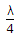# Electronics and Communication Engineering - Microwave Communication

Exercise : Microwave Communication - Section 6
26.
To ensure that only dominant TE10 mode propagates in a rectangular waveguide, the operating frequency
must be more than cutoff frequency of TE10 mode
must be less than cutoff frequency of TE20 mode
must be more than cutoff frequency of TE10 mode and less than cutoff frequency of TE20 mode
must be less than cutoff frequency of TE10 mode
Explanation:
No answer description is available. Let's discuss.

27.
In a microwave power measurement using bolometer, the principle of working is variation of
inductance with absorption of power
resistance with absorption of power
capacitance with absorption of power
cavity dimension with heat generated by power
Explanation:
No answer description is available. Let's discuss.

28.
Z0 = (Zoc Zsc)0.5
True
False
Explanation:
No answer description is available. Let's discuss.

29.
A microwave junction is matched at all ports if the S matrix
has all diagonal elements zero
has all diagonal elements equal but not zero
has all diagonal elements complex
is Hermitian
Explanation:
No answer description is available. Let's discuss.

30.

Assertion (A): A line of length slightly more thanand open circuited at far end is equivalent to an inductive reactance.

Reason (R): A line of length slightly more thanand short circuited at far end behaves as a capacitive reactance.

Both A and R are correct and R is correct explanation of A
Both A and R are correct but R is not correct explanation of A
A is correct but R is wrong
A is wrong but R is correct